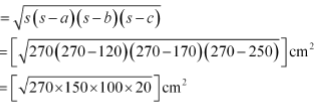Guru

# Sides of a triangle are in the ratio of 12 : 17 : 25 and its perimeter is 540cm. Find its area. Q.5

• 0

Please guide me the best way for solving the question of class 9th math of Heron’s Formula chapter of exercise 12.1 of math of question no.5 What is the best way for solving this question, please guide me Sides of a triangle are in the ratio of 12 : 17 : 25 and its perimeter is 540cm. Find its area.

Share

1. The ratio of the sides of the triangle are given as 12 : 17 : 25

Now, let the common ratio between the sides of the triangle be “x”

∴ The sides are 12x, 17x and 25x

It is also given that the perimeter of the triangle = 540 cm

12x+17x+25x = 540 cm

54x = 540cm

So, x = 10

Now, the sides of triangle are 120 cm, 170 cm, 250 cm.

So, the semi perimeter of the triangle (s) = 540/2 = 270 cm

Using Heron’s formula,

Area of the triangle= 9000 cm2

• 0